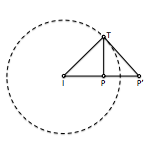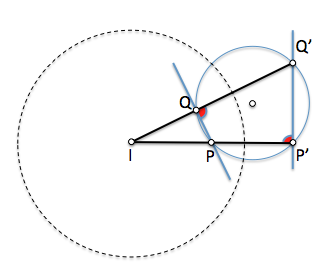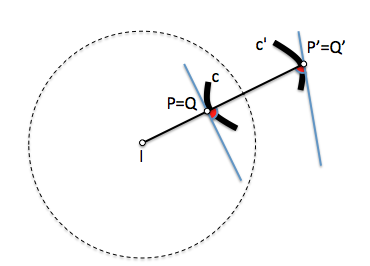# Metric geometry : Investment in the planeThe investment is a transformation homográfica retaining the angular relationships (is in accordance).

Its main application is the determination of geometry problems with angular conditions including solving are tangents exercises.

It is based on the concepts of power; is a transformation involutiva elements that can be double in cases of positive power.

## Transform definition

The investment is a processing center. This means that transformed point and are aligned with the center of inversion, analogously to the transformation known as homotecia.

The relationship between the relative positions of each point and the center of transformed investment compared based on the concept power.

Given a center “I“, and a pair of inverse points “P” and “P’“, the product of the distances these points the investment center is constant and is called Power inversion.

IP * IP’ = IQ * IQ’ = K*K = \$latex K^2\$

If the power of investment is positive, point and are transformed to the same side of the center of inversion. Los puntos is surprisingly encuentran the distancia K del Centro sound dobles. The circumference of the root radius of the power, valor K, es doble y de puntos dobles, denominating circumference autoinversión.

If the power is negative the center of inversion is located between each point and its transformed. The circumference is self-inverting stunt double but no points.

## Inversion of elements

Investment study points along with four possible cases of transformation, two for the line and two for the circumference, in which the center of inversion can be in any position on the geometric element or located on it.

• Straight containing investment center
• Lines that do not contain the center of inversion
• Circles containing the inversion center
• Circles that contain the investment center

## Inversion Point

Investing points can be solved by Power constructions or called theorems of leg and height.

### Investment positive power

In this case one of the points inside the circumference is self-inverting and the other outer ( or doubles and are on her), but at the same side with respect to I. We can apply the Theorem catheter using self inverting circumference as seen in Figure.

Los Power Concepts allow us to ensure that two points and their inverses are concíclicos (are on the same circumference which is double in the investment and cut orthogonally to the same).

### Reversing negative power

A negative inversion can be achieved by a positive equal power (modulo) March una simetría quintal. Applying height theorem determine pairs of inverse points.

The diametrical points on the circumference are inverse self-inverting.

## Investment center lines containing investment

This case is simpler since, by the definition of the transformation, the inverse of each point is aligned with this point and the center of inversion and therefore the inverse of the line, if it contains the investment center, is the line itself.

## Investment circles containing the inversion center

These two cases can be studied together because the transformation is involutive and, as discussed, the Invesa of a line that contains the center of inversion is a circle that contains it and vice versa.

As two points and their inverses are concíclicos the lines joining two points and their inverses are linking antiparallel of lines connecting each point supports and its inverse (two to two the same angle). In the figure the line PQ form an angle alpha with the straight QQ’ which is identical to the straight P'Q’ PP '.

By investing a circle passing through the center of inversion and a point P, its inverse will pass the transformed P '. If we invest another point Q on Q’ we see that the Q angle shown in figure should be straight to be inscribed in a semicircle. Consequently, the segment P'Q’ should form a right angle with the line PP’ and is required to be in the line c '. Repeating this operation for the infinite points of the circle will get straight c’

Thus:

The inverse of a circle passing through the center of inversion is a line that does not pass, direction perpendicular to the diameter that contains the inversion center.

As the transformation is involutive:

The inverse of a line not passing through the center of inversion is a circle centered on the perpendicular from the center of that line investment.

## Investment circles that do not contain the center of inversion

By studying the transformation by homotecia have seen that two coplanar circles can be related by two different centers. In the figure shows the center I establishing a postive dilation ratio in which T and T’ son homólogos, like P and Q’ either Q and P’. The reason therefore is homothetic:

IT / IT’ = IP / IQ’ = IQ / IP’ = Kh

On the other hand, power point I about the circumference c this is:

W = IP * IQ

Dividing the power ratio by the homothetic:

W / Kh = IQ * IQ’ = cte

We see that the two circles are inverse center I and power W / Kh

Therefore:

The inverse of a circle that does not pass through the center of inversion is another circle, being the center of inversion center that relates homotecia.

When the center of dilation is outside the circles of power value is positive, so that the sign inversion of the power corresponding to the centers homotecia. Sin embargo, if the center of dilation is interior to the circles, the sign is reversed.

When the homothetic center is located on the circumference, to be the zero power, can not be considered to be investment.

Note that although the centers of the circles are homologous, are not inverses.

Reverse O’ center O a circumference c that does not pass through the center of inversion is the walk from the center of polar reversal for reverse circumference c’.

## Conformity of transformation

A transformation is satisfactory if the angle two elements is the same as the elements forming the transformed. Investment is a conformal transformation so it is very useful in solving problems with angular conditions.

The antiparallelism between the lines joining two points and their inverses, for which join each with its inverse is the basis of the demonstration.The lines connecting four points of a circle, the sound of the antiparallel

C Assuming a curve through two points P and Q. Segment PQ is a chord of the curve. Borderline, when we match the points P and Q, rope becomes tangent to the curve at that:

The angle between the tangent to a curve at a point P with the line containing the point and its inverse, is the same as that forming the inverse tangent of the curve.A curve and its inverse form the same angle with the line joining each pair of inverse points

Apply themselves to the curves, stored respectively at the angle tangents we conclude that:

The angle between two curves is the same as that formed by its reverse curve, thus investment is a conformal.

The application to problem solving can be done in two conceptually different ways:

• Simplifying the problem data.
• Simplifying the solution sought.

We will see a new entry in-depth discussion on these two analysis models, aplicándolos a problem angularidad.

Metric geometry